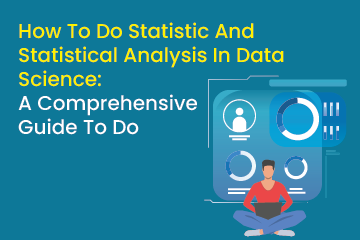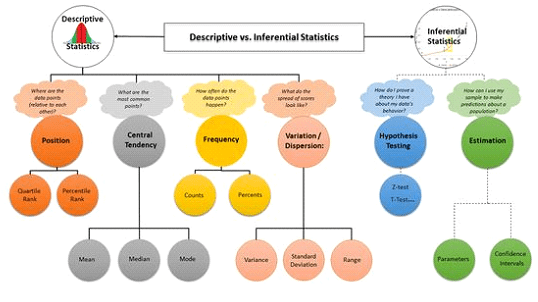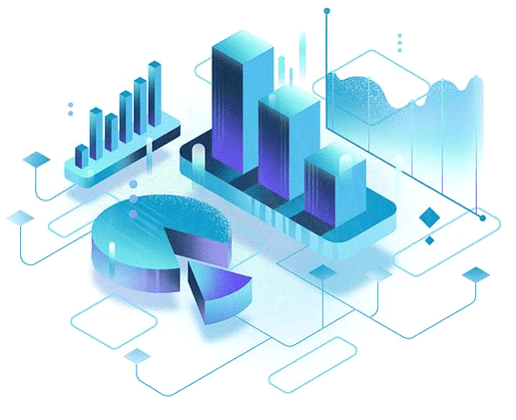Home / Blog / Data Science / How To Do Statistic And Statistical Analysis In Data Science: A Comprehensive Guide To Do

# How To Do Statistic And Statistical Analysis In Data Science: A Comprehensive Guide To Do

• February 17, 2023
• 3193
• 92### Meet the Author : Mr. Bharani Kumar

Bharani Kumar Depuru is a well known IT personality from Hyderabad. He is the Founder and Director of Innodatatics Pvt Ltd and 360DigiTMG. Bharani Kumar is an IIT and ISB alumni with more than 17 years of experience, he held prominent positions in the IT elites like HSBC, ITC Infotech, Infosys, and Deloitte. He is a prevalent IT consultant specializing in Industrial Revolution 4.0 implementation, Data Analytics practice setup, Artificial Intelligence, Big Data Analytics, Industrial IoT, Business Intelligence and Business Management. Bharani Kumar is also the chief trainer at 360DigiTMG with more than Ten years of experience and has been making the IT transition journey easy for his students. 360DigiTMG is at the forefront of delivering quality education, thereby bridging the gap between academia and industry.

With technological advancements, it has become easier to gather, organize, manipulate, and communicate data, but as it accumulates, it becomes more challenging to organize, manipulate, and communicate it. According to many scholars, data science is essential for comprehending vast amounts of data. Therefore, you will need to add the necessary skill, namely statistics for data science and the corresponding statistical methods, if you want to make significant, high-quality, risk-controlled decisions based on conclusions about the world outside the currently available data.Become a Data Scientist with 360DigiTMG Data Science course in Hyderabad Get trained by the alumni from IIT, IIM, and ISB.

## What is Statistics?

In statistics, we use mathematical tools and approaches to answer crucial questions about data. It is split into the following two groups:

Descriptive statistics: this provides ways to summarize data by turning unprocessed observations into understandable data that is simple to communicate.

Inferential statistics provides techniques for analyzing experiments carried out on small data samples and drawing conclusions that apply to the entire population (entire domain).

Are you looking to become a Data Scientist? Go through 360DigiTMG's PG Diploma in Data Science and Artificial Intelligence!.

## What is Statistical Analysis?

The purpose of statistical analysis is to discover trends and patterns in data by gathering and analyzing it. The purpose of this technique is to eliminate bias in the evaluation of data by using numerical analysis. This method is beneficial for gathering research interpretations, creating statistical models, and organizing surveys and studies.Statistical analysis is a scientific instrument that assists in collecting and analyzing large amounts of data. In addition, statistical analysis is a data analysis tool that aids in creating meaningful conclusions from unstructured, unprocessed data.

You can reach conclusions through statistical analysis, which aids decision-making and assists organizations in forecasting the future based on historical trends. It is the science of gathering, examining, and presenting data to spot trends and patterns. Statistical analysis involves working with numbers, which corporations and other institutions use to analyze data to produce useful information.## Statistics for Data Science Terminology:

• When working with Statistics for Data Science, it is important to understand a few basic statistical terminologies.
• The group of sources from whom must gather the data is known as the population.
• Samples are portions of the population.
• Any trait, quantity, or number that can be measured or counted is a variable. A data item is the another name for a variable.
• A statistical parameter, often known as a population parameter, is a variable that indexes a family of probability distributions—for instance, a population's mean, median, etc.

Want to learn more about data science? Enroll in the Best Data Science courses in Chennai to do so.

## Level of Measurement in Statistical Analysis

In statistics, the level of measurement is a categorization that represents the connection between the values of a variable.

Four basic degrees of measuring exist. And these are:

• Nominal Scale
• Ordinal Scale
• Interval Scale
• Ratio Scale
• Nominal Scale: Due to the data having names and labels, this scale contains the least amount of information. It can be classified using it. You cannot use nominal data for mathematical operations since the options have no numerical value (numbers associated with the names you can only use as tags). Example: What country do you represent? Korea, Japan, and India.
• Ordinal Scale: In contrast to the nominal scale, the ordinal scale contains more data since, in addition to the labels, it also includes order and direction.
Example: Three income levels: high, medium, and poor.
• Interval Scale: This scale is known as a numerical scale. The interval scale contains more information than the nominal and ordinal scales. We are aware of the distinction between the two variables in addition to the order (interval indicates the distance between two entities).
You can use the data's mean, median, and mode to describe it.
Example: Income, temperature, etc.
• Ratio Scale: This scale provides the most significant details regarding the data. The ratio scale is the only one that supports a true zero point among the other three scales. Therefore, the combination of the nominal, ordinal, and interval scales is known as the ratio scale.
Example: Your current size, height, etc.## Practical Statistical Analytics Learning Tips

Most colleges have created statistics course curricula to gauge a student's ability to cram. However, instead of emphasizing how to use these techniques to address real-world issues, they test students' ability to solve equations, define terminology, and recognize charts derived from them.

However, aspiring practitioners should adhere to a sequential process of learning and applying statistical techniques to various issues using executable Python code.

Let's take a deeper look at the two primary methods for studying statistics:

⦁ Top-down strategy:

Consider the scenario in which you create an experiment to compare the effectiveness of two product features. This function is to improve user interaction with an online portal.

A top-down strategy involves first learning more about the issue. Then, after establishing the goal, you can learn how to use the right statistical techniques. It keeps you interested and provides a better opportunity for applied learning.

⦁ Bottom-up strategy:

Most colleges and online courses teach statistics in this manner. The emphasis is on understanding the theoretical ideas expressed mathematically, their origins, and practical applications.

There are better ways to study applied statistics for those like myself who become bored with theoretical learning. But, unfortunately, it turns the subject dull and gloomy and removes any direct connection to issue-solving by making it too meta.

## Statistical Analysis Methods

Although there are many ways to analyze data, the five most common and widely used statistical analysis techniques are listed below:

⦁ Mean:

An important approach to statistical analysis is the mean or average mean. The mean, which is fairly easy to calculate, determines the overall trend of the data. You can determine the mean by adding all the values in the data set, then dividing it by total number of data points. Unfortunately, despite the simplicity of calculation and its advantages, using the mean as the main statistical indicator is not good because doing so can lead to erroneous judgments.

⦁ Standard Deviation:

Another very popular statistical tool or technique is the standard deviation. It examines how far each data point deviates from the overall data set mean. You can use it to determine whether or not the research findings are generalizable.

⦁ Regression:

Regression is a statistical technique that aids in establishing the causal connection between the variables. It establishes how a dependent variable and an independent variable are related. You can predict future trends and events using it.

⦁ Testing Hypotheses:

You can put a conclusion or argument to the test via hypothesis testing against a set of facts. The research's initial premise, the hypothesis, may prove to be true or erroneous depending on the study's findings.

⦁ Sample Size Calculation:

A technique used to extract a sample from the complete population representative of the population is sample size determination or data sampling. When the population is exceedingly huge, this strategy is employed. You can select from various data collection methods, including convenience, random, and snowball sampling.

Earn yourself a promising career in data science by enrolling in the Data Science Classes in Pune offered by 360DigiTMG.

## How to do Descriptive Statistical Analysis

Data is represented based on some central tendency when we visualize it using graphs, such as histograms, line plots, etc. For statistical analysis, central tendency measurements such as mean, median, or measures of the spread, etc. Let's use an example to talk about the various statistical metrics to comprehend them better.

Here is an example automobile data set with the variables:

Terminologies used in cars:

⦁ Mileage per Gallon (mpg)

⦁ Cylinder Type (cyl)

⦁ Displacement (disp)

⦁ Horse Power (hp)

⦁ Real Axle Ratio (drat)

Cars mpg cyl disp hp drat
C1 22 4 130 95 3.6
C2 21.5 4 160 105 3.9
C3 23 6 140 110 3.75
C4 23 4 150 96 3.8
C5 22 4 107 98 3.95
C6 22.3 6 110 110 4
C7 22.3 4 107 106 4
C8 22 6 107 108 4

## Measures of the Center:

⦁ Mean: The term "Mean" refers to the average value across all sampled values.

⦁ Median: The Median measures the sample set's central value.

⦁ Mode: The term "Mode" refers to the most recurrent value in the sample set.

A descriptive analysis determines the means, standard deviations, minimums, and maximums of each variable in the sample.

We will examine and compute the average of all data to determine the mean or average horsepower of the cars among the population of cars. In this instance, we'll divide the entire number of cars by the amount of their horsepower:

Mean = (95+105+110+96+98+110+106+108)/8 = 103.5

We can arrange the mpg numbers in ascending or descending order and then select the middle value to determine the average mpg among the population of cars. We have eight values in this instance, making it an even entry. We must therefore calculate the average of the two middle values.

The mpg for 8 cars: 21.5,22,22,22,22.3,22.3,23,23

Median = (22+22.3 )/2 = 22.15

We will look at the value that is repeated the most frequently to determine the most prevalent cylinder type among the population of cars. As seen below, the cylinders are available in two values, 4 and 6. Look at the data set to find that 4 appears the most frequently. 4 is hence our Mode.

We also have spread measurements, including the following measures and the center measure.

⦁ Range: It measures the degree of dispersion between values in a data set.

⦁ (IQR) Inter Quartile Range: It is known as a measure of variability based on quartilizing a data set.

⦁ Variance: It refers to how much a random variable deviates from the value that is predicted. It involves calculating deviation squares.

⦁ The difference between each element and the mean is known as the deviation.

⦁ The average of squared deviations represents Population variation.

⦁ The average squared deviations from the mean are known as sample variance.

⦁ Standard Deviation: This term refers to measuring a set of data's dispersion from its mean.

## How to do Inferential Statistical Analysis

Hypothesis testing is a technique used by statisticians to determine whether a hypothesis is accepted or rejected explicitly. In addition, an inferential statistical approach called hypothesis testing is used to assess if there is sufficient evidence in a data sample to conclude that a particular condition is true for the entire population.

We select a random sample and examine the sample's features to determine the characteristics of the general population. Finally, we do a test to determine whether the indicated conclusion truly depicts the population. The percentage value we obtain from the hypothesis determines whether or not we should accept it.

Think about four girls caught skipping class: Annie, Jessie, Barbie, and Chitra. As a punishment, they were instructed to stay in class and clean their classroom.

Jessie decided that the four of them would clean their classroom alternately. She devised a scheme in which each of their names would be written on chits and placed in a bowl. Each day, they had to choose a name out of a bowl, and that individual was responsible for cleaning the class.

After three days, everyone's name was called, but not Jessie's! What is the likelihood that Jessie isn't lying if this incident is totally random and impartial?

Let's first determine the likelihood that Jessie won't be selected for a day:

P(Jessie not picked for a day) = 3/4 = 75%

75% is a rather high probability in this case. Now, the probability reduces to 42% if Jessie is not chosen three days in a row%

P(Jessie not picked for 3 days) = 3/4 ×3/4× 3/4 = 0.42 (approx)

Consider the following scenario: Jessie is not picked for 12 consecutive days! The probability decreases to 3.2%. The probability of Jessie cheating is, therefore, quite high.

P(Jessie not picked for 12 days) = (3/4) ^12 = 0.032

Statisticians define a threshold value, which they then use to conclude. In light of the circumstance above, if the threshold value is set at 5%, it would mean that John is absconding from incarceration by cheating if the chance is lower than 5%. However, if the chance is higher than the cutoff point, Jessie is lucky, and his name isn't chosen.

Two key notions are generated from probability and hypothesis testing, namely:

Null Hypothesis: The outcome is identical to the prediction.

Alternative Hypothesis: The outcome contradicts the presumption.

Therefore, in our example, if an event occurs with a probability of less than 5%, it isn't very objective and supports the alternative hypothesis.

## Become Proficient in Statistics Analysis Today with 360digiTMG:

You may execute statistical analysis and data analysis successfully and efficiently using artificial intelligence (AI). Check out this incredible statistical analysis course offered by 360digiTMG in partnership with IBM if you are a science whiz captivated by AI's use in statistical analysis. This Statistical Analytics course will teach you that all you need to know about statistical analysis in data science and is one of the most popular courses due to its extensive syllabus and real-world projects.

### Data Analyst Courses in Other Locations